Courses

# Test: Comprehension Based Questions: The d- and f-Block Elements & Coordination Compounds

## 13 Questions MCQ Test Class 12 Chemistry 35 Years JEE Mains &Advance Past yr Paper | Test: Comprehension Based Questions: The d- and f-Block Elements & Coordination Compounds

Description
This mock test of Test: Comprehension Based Questions: The d- and f-Block Elements & Coordination Compounds for JEE helps you for every JEE entrance exam. This contains 13 Multiple Choice Questions for JEE Test: Comprehension Based Questions: The d- and f-Block Elements & Coordination Compounds (mcq) to study with solutions a complete question bank. The solved questions answers in this Test: Comprehension Based Questions: The d- and f-Block Elements & Coordination Compounds quiz give you a good mix of easy questions and tough questions. JEE students definitely take this Test: Comprehension Based Questions: The d- and f-Block Elements & Coordination Compounds exercise for a better result in the exam. You can find other Test: Comprehension Based Questions: The d- and f-Block Elements & Coordination Compounds extra questions, long questions & short questions for JEE on EduRev as well by searching above.
QUESTION: 1

### PASSAGE 1 The coordination number of Ni2+ is 4. NiCl2 + KCN (excess) → A (cyano complex) NiCl2 + Conc . HCl (excess) → B (chloro complex) Q.The IUPAC name of A and B are

Solution: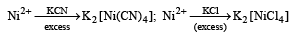QUESTION: 2

### PASSAGE 1 The coordination number of Ni2+ is 4. NiCl2 + KCN (excess) → A (cyano complex) NiCl2 + Conc . HCl (excess) → B (chloro complex) Q. Predict the magnetic nature of A and B

Solution: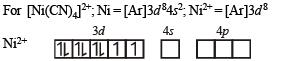However, CN is a strong field ligand so it forces the 3d electrons to pair up and hence the effective configuration in this case will be Ni2+ in presence of CN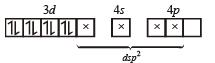Thus [Ni (CN)4]2– exhibits dsp2 hybridization and square planar shape. Since here number of unpaired electrons is zero the complex will be diamagnetic.
In case of [NiCl4]2–, Cl is a weak field ligand, so the effective configuration of Ni2+ in this complex will be as follows : Ni2+ in presence of Cl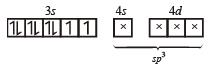So here Ni2+ is sp3 hybridised and thus tetrahedral in shape. Since the complex has two unpaired electrons, it will be paramagnetic.

QUESTION: 3

### PASSAGE 1 The coordination number of Ni2+ is 4. NiCl2 + KCN (excess) → A (cyano complex) NiCl2 + Conc . HCl (excess) → B (chloro complex) Q. The hybridization of A and B are

Solution:

Discussed above.

QUESTION: 4

PASSAGE 2

Copper is the most noble of the first row transition metals and occurs in small deposits in several countries. Ores of copper include chalcanthite (CuSO4.5H2O), atacamite (Cu2Cl(OH)3), cuprite (Cu2O), copper glance (Cu2S) and malachite (Cu2(OH)2CO3). However, 80% of the world copper production comes from the ore chalcopyrite (CuFeS2). The extraction of copper from chalcopyrite involves partial roasting, removal of iron and self-reduction.

Q. Partial roasting of chalcopyrite produces

Solution: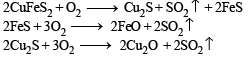QUESTION: 5

PASSAGE 2

Copper is the most noble of the first row transition metals and occurs in small deposits in several countries. Ores of copper include chalcanthite (CuSO4.5H2O), atacamite (Cu2Cl(OH)3), cuprite (Cu2O), copper glance (Cu2S) and malachite (Cu2(OH)2CO3). However, 80% of the world copper production comes from the ore chalcopyrite (CuFeS2). The extraction of copper from chalcopyrite involves partial roasting, removal of iron and self-reduction.

Q. Iron is removed from chalcopyrite as

Solution:

FeO + SiO2→ FeSiO3

QUESTION: 6

PASSAGE 2

Copper is the most noble of the first row transition metals and occurs in small deposits in several countries. Ores of copper include chalcanthite (CuSO4.5H2O), atacamite (Cu2Cl(OH)3), cuprite (Cu2O), copper glance (Cu2S) and malachite (Cu2(OH)2CO3). However, 80% of the world copper production comes from the ore chalcopyrite (CuFeS2). The extraction of copper from chalcopyrite involves partial roasting, removal of iron and self-reduction.

Q. In self-reduction, the reducing species is

Solution:

Cu2S + 2Cu2O → 6Cu + SO2

The reducing species is the one which gets oxidised.
So, it is S2– ion getting oxidised to S4+.

QUESTION: 7

PASSAGE 3

When a metal rod M is dipped in to an aqueous colour less concentrated solution of compound N, the solution turns light blue. Addition of aqueous NaCl to the blue solution gives a white precipitate O. Addition of aqueous NH3 dissolves O and gives an intense blue solution.

Q. The metal rod M is

Solution:
QUESTION: 8

PASSAGE 3

When a metal rod M is dipped in to an aqueous colour less concentrated solution of compound N, the solution turns light blue. Addition of aqueous NaCl to the blue solution gives a white precipitate O. Addition of aqueous NH3 dissolves O and gives an intense blue solution.

Q. The final solution contains

Solution: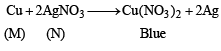QUESTION: 9

PASSAGE 3

When a metal rod M is dipped in to an aqueous colour less concentrated solution of compound N, the solution turns light blue. Addition of aqueous NaCl to the blue solution gives a white precipitate O. Addition of aqueous NH3 dissolves O and gives an intense blue solution.

Q. The compound N is

Solution:
QUESTION: 10

Read the following statement-1(Asseration/Statement) and Statement -2 (Reason/Explanation) and answer as per the options given below :

Q.

Statement-1 : To a solution of potassium chromate if a strong acid is added it changes its colour from yellow to orange.
Statement-2 : The colour change is due to the oxidation of potassium chromate.

Solution:

The statement is correct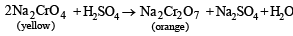Oxidation state of Cr in Na2CrO4 and Na2Cr2O7 is +6, i.e. no change in O.S. So explanation is wrong

QUESTION: 11

Statement-1 : Zn2+ is diamagnetic.
Statement-2 : Two electrons are lost from 4s orbital to form Zn2+.

Solution: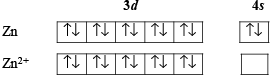Zn2+ is diamagnetic because of absence of unpaired electrons.

QUESTION: 12

Statement-1 : The geometrical isomers of the complex [M(NH3)4Cl2] are optically inactive. and

Statement-2 : Both geometrical isomers of the complex [M(NH3)4Cl2] possess axis of symmetry.

Solution:

The geometrical isomers of [M(NH3)4Cl2] can be represented as follows:-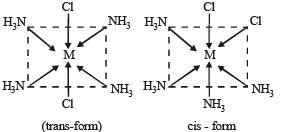These isomers are optically inactive and they posses axis of symmetry.
Both the statements are thus true. Out of two possible answers i.e. option (a) and (b) option (b) is correct as the statement 2 is not a correct explanation of statement 1.
For a molecule to be optically active it should not possess alternate axis of symmetry.

QUESTION: 13

Statement-1 : [Fe(H2O)5NO]SO4 is paramagnetic. and

Statement-2 : The Fe in [Fe(H2O)5NO]SO4 has three unpaired electrons.

Solution:

In [Fe(H2O)5NO] SO4, Let the oxidation state of Fe be x. Then for [Fe(H2O)5 NO]2–,
x + 1 = + 2 or x = + 2 – 1 = + 1
Hence in this complex the oxidation state of Fe is + 1 Electronic configuration of Fe+ can be represented as Fe+ = 1s22s22p63s23p63d7. This unexpected configuration is due to presence of strong ligand field.
Due to which 1 electron from 4s1 gets shifted to 3d– orbitals.
The 3d7 electrons in five 3d– orbitals can be shown as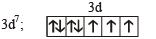In it we find 3 unpaired electrons.
Because of the presence of unpaired electrons the complex is paramagnetic i.e. statement 1 is true.

As is clear from above there are three unpaired electrons in this complex i.e. statement 2 is true.
Since paramagnetic behaviour is due to presence of unpaired electrons in it so statement 2 is correct explanation of statement 1.
In view of the above facts the correct answer is option (a)